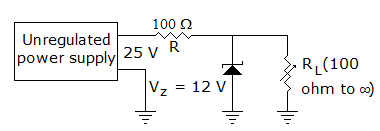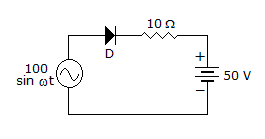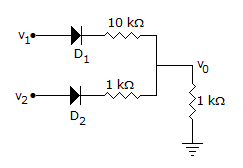# Electronics and Communication Engineering - Analog Electronics

11.

In figure the zener currentA. is always 130 mA B. may vary between 10 mA and 130 mA C. may vary between 60 mA and 130 mA D. is always equal to 65 mA

Explanation:

When IL = 0, Zener current = 130 mA, when IL = 120 mA, zener current (t = 10 mA).

12.

In a commercially available good power supply the voltage regulation is about

 A. 1% B. 5% C. 10% D. 20%

Explanation:

A good power supply does not allow output voltage to vary by more than 1% .

13.

In the circuit of figure the diodeA. will conduct as the whole cycle B. will conduct in the positive half cycle C. will not conduct D. will conduct from 30° to 150° in the positive half cycle

Explanation:

Diode will always be reverse biased.

14.

The most commonly used bias in BJT amplifier circuits is

 A. voltage divider bias B. emitter bias C. collector bias D. collector feedback bias

Explanation:

Voltage divider bias stabilizes IC.

15.

In the circuit of figure, v1 = v2 = 10 V. ThenA. I1 = I2 B. I1 > I2 C. I2 > I1 D. I1 = 0